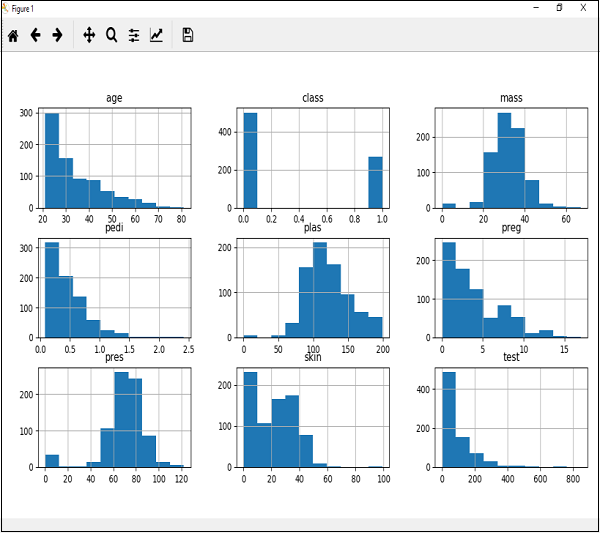# Machine Learning with Python - Histograms

Histograms group the data in bins and is the fastest way to get idea about the distribution of each attribute in dataset. The following are some of the characteristics of histograms −

• It provides us a count of the number of observations in each bin created for visualization.

• From the shape of the bin, we can easily observe the distribution i.e. weather it is Gaussian, skewed or exponential.

• Histograms also help us to see possible outliers.

## Example

The code shown below is an example of Python script creating the histogram of the attributes of Pima Indian Diabetes dataset. Here, we will be using hist() function on Pandas DataFrame to generate histograms and matplotlib for ploting them.

from matplotlib import pyplot
path = r"C:\pima-indians-diabetes.csv"
names = ['preg', 'plas', 'pres', 'skin', 'test', 'mass', 'pedi', 'age', 'class']
data.hist()
pyplot.show()


## OutputThe above output shows that it created the histogram for each attribute in the dataset. From this, we can observe that perhaps age, pedi and test attribute may have exponential distribution while mass and plas have Gaussian distribution.

machine_learning_with_python_understanding_data_with_visualization.htm